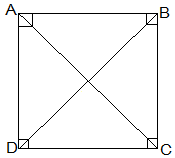# ABCD is a rectangle in which diagonal AC bisects ∠A as well as ∠C. Show that: (i) ABCD is a square (ii) Diagonal BD bisects ∠B as well as ∠D.A rectangle ABCD such that AC bisects ∠A as well as ∠C, so

∠BAC = ∠DAC and,

∠DCA = ∠BCA ………………………………(1)

(i) ABCD is a square

As we know that every rectangle is a parallelogram.

ABCD is a parallelogram.

∠BCA = ∠DAC …………………….(2) [ Alternate interior angles are equal]

From (1) and (2), we have

∠DCA= ∠DAC……………………….(3)

In ∆ABC, ∠DCA= ∠DAC then,

CD = DA [Sides opposite to equal angles of a ∆ are equal]

Similarly, AB = BC

So, ABCD is a rectangle having adjacent sides equal.

ABCD is a square.

(ii) Diagonal BD bisects ∠B as well as ∠D.

Since, ABCD is a square

AB = BC = CD = DA

so, In ∆ABD, as AB = AD

∠ABD = ∠ADB [Angles opposite to equal sides of a ∆ are equal]……………………..(1)

Similarly, ∠CBD = ∠CDB…………………..(2)

∠CBD = ∠ADB [Alternate interior angles are equal]………………(3)

From (1) and (3)

∠CBD = ∠ABD

From (2) and (3)

Thus, BD bisects ∠B as well as ∠D.